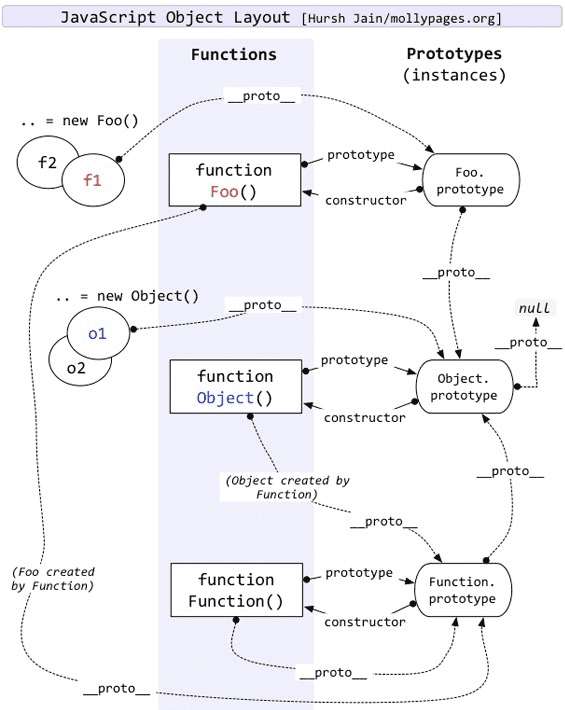# JavaScript 中的始皇 ​

## 前言 ​javascript
``````Object instanceof Object // true
Function instanceof Function // true
Object instanceof Function // true
Function instanceof Object // true``````

## 正文 ​

javascript
``````String instanceof Function // true
Array instanceof Function // true
Function instanceof Function // true
Object instanceof Function // true``````javascript
``Function.__proto__ === Function.prototype``javascript
``````String.prototype.__proto__ === Object.prototype // true
Number.prototype.__proto__ === Object.prototype // true
Array.prototype.__proto__ === Object.prototype // true
Function.prototype.__proto__ === Object.prototype // true
Object.prototype.__proto__ === null // true

var obj = {};
var arr = [];
function func() {}
obj.__proto__ ===  Object.prototype // true
arr.__proto__.__proto__ === Object.prototype // true
// arr.__proto__ 指向 Array.prototype
func.__proto__.__proto__ === Object.prototype // true
// func.__proto__ 指向 Function.prototype``````Object.prototype 的原型之母，任何原型都源自它

Function.prototype 是构造函数之母，任何构造函数都由它创建，包括它自己

javascript
``````Object instanceof Object // true
// Object 由 Function.prototype 创建，Function.prototype 的原型对象指向 Object.prototype
Function instanceof Function // true
// Function 由 Function.prototype 创建
Object instanceof Function // true
// Object 由 Function.prototype 创建
Function instanceof Object // true
// Function 由 Function.prototype 创建，Function.prototype 的原型对象指向Object.prototype``````